# Soft limiter for oscillator circuits uses emitter-degenerated differential pair

## Texas Instruments TL081

,

EDN

Most oscillator circuits include a nonlinear amplitude control that sustains oscillations at a desired amplitude with minimum output distortion. One approach uses the output sinusoid's amplitude to control a circuit element's resistance, such as that of a JFET operating in its triode-characteristics region. Another control method uses a limiter circuit that allows oscillations to grow until their amplitude reaches the limiter's threshold level. When the limiter operates, the output's amplitude remains constant. To minimize nonlinear distortion and output clipping, the limiter should exhibit a "soft" characteristic.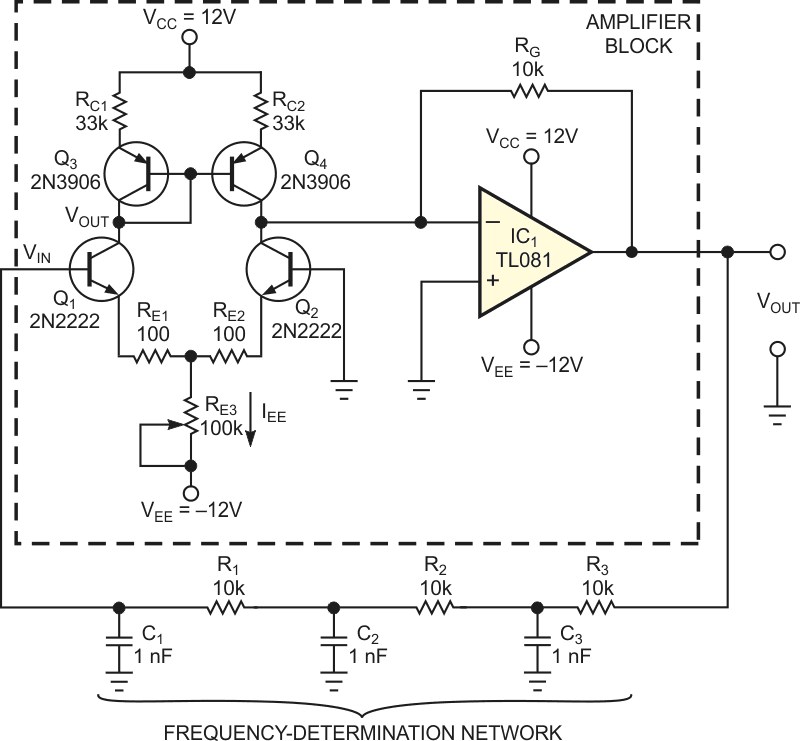Figure 1. A phase-shift RC-oscillator circuit uses an emitter-coupled amplitude limiter.

Based on a waveform shaper that imposes a soft limitation or saturation characteristic, the circuit in Figure 1 comprises a simple RC (resistor-capacitor)-ladder phase-shift oscillator and an amplitude-control limiter circuit. R1, R2, and R3 have values of 10 kΩ each, and C1, C2, and C3 have values of 1 nF each. The following equation defines output voltage VOUT's frequency, fO.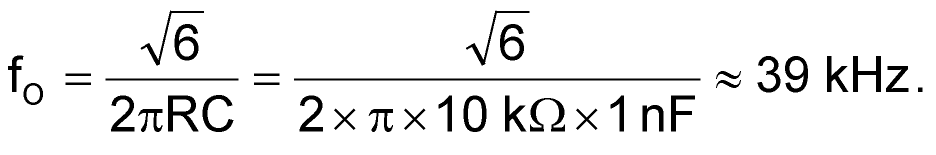The inverting-amplifier block in Figure 1 comprises transistors Q1 and Q2, a differential pair that presents a nonlinear-transfer characteristic, plus an IVC (current-to-voltage converter) based on operational amplifier IC1. For oscillation to occur, the inverting amplifier's gain magnitude must exceed 29. Selection of appropriate values of bias current, IEE; the transistor pair's emitter-degeneration resistances, RE1 and RE2; and RE3 produces the amplifier's nonlinear-transfer characteristic, VOUT versus VIN (Figure 2).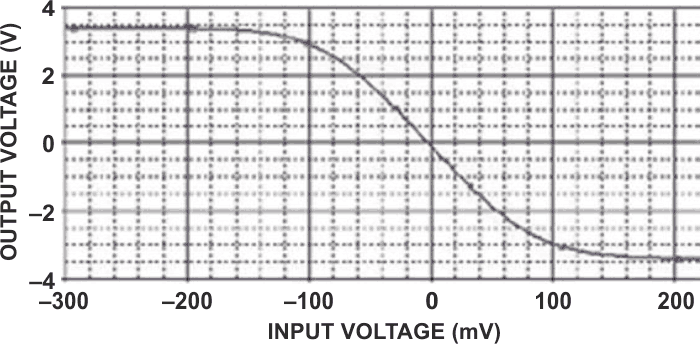Figure 2. The transfer-characteristic output voltage versus input voltage for the nonlinear amplifier shows a gradual onset of limiting at approximately 100-mV input.

A small input voltage produces a nearly linear-amplifier-transfer characteristic. However, large values of input voltage drive Q1 and Q2 into their nonlinear region, reducing the amplifier's gain and introducing a gradual bend in the transfer characteristic. A current mirror comprising Q3 and Q4 converts the shaping circuit's output to a single-ended current, which operational amplifier IC1 converts to an output voltage. In the prototype circuit, calibration trimmer RE3 has a value of approximately 33 kΩ. Figure 3 shows the oscillator's output voltage for the component values in Figure 1, and Figure 4 shows the sinusoidal output's spectral purity.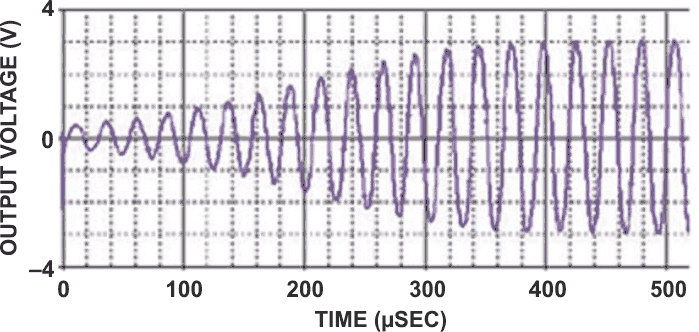Figure 3. For the component values in Figure 1, the oscillator’s output voltage reaches full amplitude in approximately 400 msec, or 15 cycles after start-up.

The nonlinear amplifier's wave-shaping action occurs independently of frequency, and this circuit offers convenience for use with variable-frequency oscillators. Note that IC1's gain-bandwidth product limits the circuit's performance. To use the limiter portion of the circuit with a noninverting amplifier, such as a Wien-bridge oscillator, apply the signal input voltage to Q2's base, and ground Q1's base.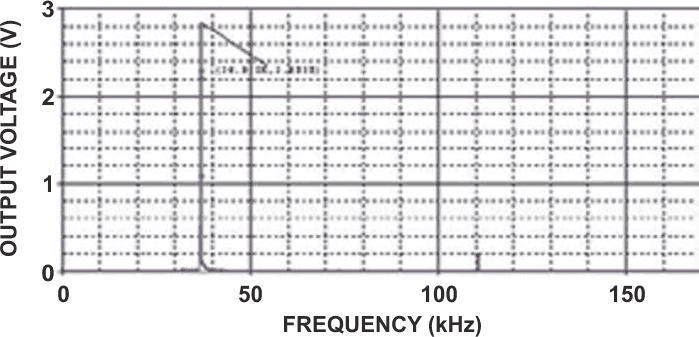Figure 4. The oscillator’s output spectrum shows only a slight amount of third-harmonic output.

EDN